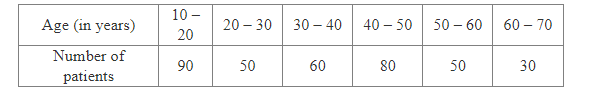# Following are the ages (in years) of 360 patients, getting medical treatment in a hospital:Question:

Following are the ages (in years) of 360 patients, getting medical treatment in a hospital:One of the patients is selected at random.
What is the probability that his age is
(i) 30 years or more but less than 40 years?
(ii) 50 years or more but less than 70 years?
(iii) 10 years or more but less than 40 years?
(iv) 10 years or more?
(v) less than 10 years?

Solution:

Total number of patients  = 360
(i) Number of patients whose age is 30 years or more but less than 40 years = 60

Let E1 be the event that the selected patient's age is in between 30 - 40.
$\therefore P$ (patient's age 30 is years or more but less than 40 years) $=P\left(E_{1}\right)=\frac{60}{360}=\frac{1}{6}$
(ii)  Number of patients whose age is 50 years or more but less than 70 years = (50 +30) = 80
Let E2 be the event that the selected patient's age is in between 50 - 70.
$\therefore P($ patient's age is 50 years or more but less than 70 years $)=P\left(E_{2}\right)=\frac{80}{360}=\frac{2}{9}$
(iii) Let Ebe the event that the selected patient is 10 years or more but less than 40 years.
Number of patients whose age is 10 years or more but less than 40 years = 90 + 50 + 60 = 200
$\therefore \mathrm{P}($ Patient's age is 10 years or more but less than 40 years $)=\mathrm{P}\left(E_{3}\right)=\frac{200}{360}=\frac{5}{9}$
(iv) Number of patients whose age is 10 years or more = 90 + 50 + 60 + 80 + 50 + 30 =  360
Let E4 be the event that the selected patient's age is 10 years or more. Then
$\therefore P($ patient's age is 10 years or more $)=P\left(E_{4}\right)=\frac{360}{360}=1$
(v) Number of patients whose age is less than 10 years = 0
Let E5 be the event that the selected patient's age is less than 0.
$\therefore P$ (patient's age is less than 10 years) $=P\left(E_{5}\right)=\frac{0}{360}=0$